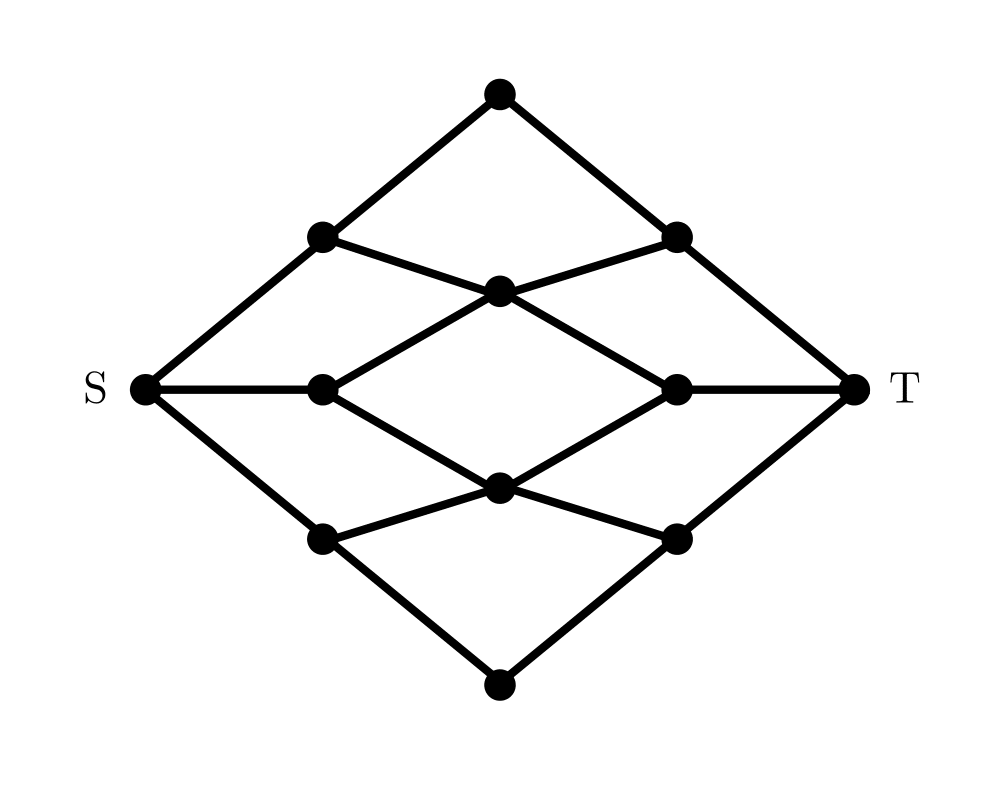# Network FlowInputOutput

Input Description: A graph $$G$$, where each edge $$(i,j)$$ has a capacity $$c_{i,j}$$. A source node $$s$$ and sink node $$t$$.
Problem: What is the maximum flow you can route from $$s$$ to $$t$$ while respecting the capacity of each edge.

Excerpt from The Algorithm Design Manual: Applications of network flow go far beyond plumbing. Finding the most cost-effective way to ship goods between a set of factories and a set of stores defines a network flow problem, as do resource-allocation problems in communications networks and a variety of scheduling problems.

The real power of network flow is that a surprising variety of linear programming problems that arise in practice can be modeled as network flow problems, and that special-purpose network flow algorithms can solve such problems much faster than general-purpose linear programming methods. Several of the graph problems we have discussed in this book can be modeled as network flow, including bipartite matching, shortest path, and edge/vertex connectivity.

Go To Main Page